# Foundations

## 1: Special Relativity

 B B A A A A A

Anyone who reads science fiction will be familiar with some of the remarkable predictions of twentieth-century physics. Time dilation, black holes, and the uncertainty principle have all been part of the SF lexicon for decades. In this series of articles I’m going to describe in detail how these phenomena arise, and along the way I hope to shed some light on the theories that underpin them: special relativity, general relativity, and quantum mechanics. The foundations of modern physics.

These articles are meant for the interested lay reader. If you can follow high school algebra and geometry, and aren’t afraid to take in a few new concepts — which is the whole point, after all — nothing here should faze you.

### Spacetime

The idea that we inhabit a four-dimensional spacetime is a very natural and intuitive one. It’s only because we take the duration of objects so much for granted that we tend to gloss over it and refer to them as three-dimensional. Since most of the Earth’s landscape changes slowly, factoring out time from our mental models and paper maps is a very pragmatic thing to do, but it’s this unchanging space that we imagine for convenience that’s the abstract mental construct, not spacetime. Spacetime is simply what we live in, all four dimensions of it.

Drawing a diagram of spacetime comes almost as naturally as making any other kind of map; every historical timeline is halfway there, and placing a timeline for Germany next to one for France, then sketching in the movement of armies between the two, is as good a spacetime diagram as anything you’ll find in particle physics. Of course, a spacetime diagram in ink on paper has only two useful dimensions, so it generally only shows time plus one dimension of space (though one more can be added, using the standard techniques for drawing three-dimensional objects). Fortunately, many problems in special relativity involve only one dimension of space; for example, a spacecraft flying from here to Sirius would almost certainly travel along a straight line.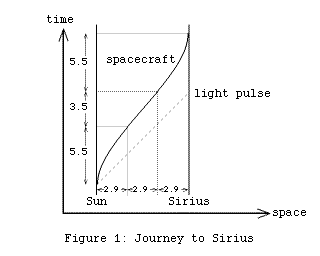Figure 1 is a spacetime diagram for such a flight. Distances are in light years and times are in years. For the sake of simplicity, the slight “proper motion” of Sirius relative to the Sun, and any orbital manoeuvres and planetary take-offs and landings by the spacecraft, are ignored. The spacecraft accelerates at the start of the journey, shuts off its engines and cruises for the middle stage, then decelerates at the end, bringing it to a halt just as it arrives. (There’s no special reason for all three stages to cover equal distances; this is just one possible flight plan of many.) Given that the distance to Sirius is almost nine light years, it’s reasonable to treat stars and spacecraft alike as mere specks, tracing out one-dimensional world lines, rather than worrying about the fact that they’re really solid objects whose histories in spacetime are four-dimensional “world hypercylinders”.

When you draw a map, you have to choose a compass direction to point “up” on the page. North is often convenient, but it’s a completely arbitrary choice, and on a house plan, say, it might be more useful to align the map so that the street frontage is horizontal. Similarly, to draw a spacetime diagram you have to choose a reference frame: you have to pick some object, such as the Sun, and treat it as fixed. The chosen object’s world line will then be vertical — it will be “moving” only in time, not space — as will the world line of any other object at a constant distance from it. So the world lines for the Sun and Sirius are vertical here because the diagram was drawn that way; it’s a matter of convenience, not a statement that the Sun is “truly motionless”, any more than north is “truly up”.

However, some reference frames are different from others. Orienting a map so that a given straight road runs vertically is one thing; arranging for a meandering river to appear as a straight line is a much harder task. If we chose the spacecraft to be the fixed point, everything we did would be complicated by the need to straighten out the curved sections of its world line when it accelerates and decelerates. To avoid this kind of complication, special relativity deals only with inertial reference frames, which take as their fixed point an object that is not accelerating. Unlike the idea of being motionless (motionless compared to what?) this condition is easily defined in the middle of interstellar space: if you’re not firing your engines, and everything in the ship is weightless, then you’re not accelerating.

Of course, we can imagine a hypothetical second spacecraft which never accelerates, but conveniently happens to match the first spacecraft’s velocity for some part of the journey — such as the entire middle stage, when the engines are shut off, or even just for an instant during the acceleration or deceleration stages. That way, we can analyse the first spacecraft’s viewpoint at any given moment, without adopting a reference frame in which it appears motionless from start to finish.

In a reference frame fixed to the Sun, the world line for the spacecraft starts out being vertical, tips over as it accelerates, has a constant slope in the cruising stage, then comes back towards vertical again as it decelerates. The world line for a pulse of light that leaves the solar system at the same time as the spacecraft is shown for comparison; it has a constant angle (45° in this diagram), because it travels at a constant velocity all the way.

To be in motion relative to the Sun means tracing out a world line at an angle to the Sun’s world line. That might sound like nothing but a novel way to describe the situation, but it’s the key to all the relativistic effects of space travel. Two people facing different directions in ordinary space see the same objects differently. Two people driving between the same two towns will travel different distances, if one takes the most direct route while the other takes a detour. In spacetime, the effects are analogous, but not quite identical, because the geometry of spacetime is not quite the same as the geometry of space.

### Rotations in Space

Despite the differences, analysing the effects of rotating your angle of view in ordinary space makes a useful rehearsal for tackling the problem in spacetime. It’s easier to deal with ordinary space, where we can rely on everyday geometrical intuition, and then the results can be carried over to spacetime with only a few small changes.First, a quick review of the geometry we’ll need. Pythagoras’s theorem says that the square of the hypotenuse (OP in Figure 2) of any right-angle triangle equals the sum of the squares of the other two sides (OQ and PQ).

 OP2 = OQ2 + PQ2

The sine of the angle marked A is equal to the ratio between the side opposite it (PQ) and the hypotenuse (OP).

 sin A = PQ / OP PQ = OP sin A

The cosine of A is equal to the ratio between the side adjacent to it (OQ) and the hypotenuse (OP).

 cos A = OQ / OP OQ = OP cos A

The tangent of A is equal to the ratio between the side opposite it (PQ), and the side adjacent to it (OQ).

 tan A = PQ / OQ = (OP sin A) / (OP cos A) = sin A / cos A

There’s a simple relationship between the sine and cosine of an angle, which comes straight from the definitions and Pythagoras’s theorem:

 (cos A)2 + (sin A)2 = (OQ / OP)2 + (PQ / OP)2 = (OQ2 + PQ2) / OP2 = OP2 / OP2 = 1

The notation “(x,y)” beside the point P is a reminder that points can be referred to by their x– and y-coordinates, written as an ordered pair. The arrow drawn from O to P is a reminder that every point can be thought of as defining a vector from the origin to the point. The advantage of dealing with vectors, rather than just points in space, is that the same geometry can then be applied to other vectors, like velocity and acceleration.

To make it easier to carry things over from Euclidean geometry to spacetime geometry, it will help to restate some of these familiar ideas in slightly different language. In both Euclidean and spacetime geometry, there’s a formula for taking two vectors and calculating a number from them which depends on the length of the vectors and the angle between them. This formula is known as the metric for the geometry. (You might also have come across it as the “dot product” of two vectors.) It’s usually written as g:

 g[(x,y),(u,w)] = xu + yw (1)

Eqn (1) defines the Euclidean metric. Eqns (2a)-(2c) demonstrate some of its properties: it’s symmetric (swapping the two vectors leaves the value unchanged), and it’s linear (its value is simply multiplied and added as shown, if you apply it to a vector that’s been multiplied by a factor, or had another vector added to it).

 g[(u,w),(x,y)] = ux + wy = xu + yw = g[(x,y),(u,w)] (2a) g[a(x,y),(u,w)] = g[(ax,ay),(u,w)] = axu + ayw = a (xu + yw) = a g[(x,y),(u,w)] (2b) g[(x,y)+(p,q),(u,w)] = g[(x+p,y+q),(u,w)] = (x+p)u + (y+q)w = (xu + yw) + (pu + qw) = g[(x,y),(u,w)] + g[(p,q),(u,w)] (2c)

Eqn (3) is just a restatement of Pythagoras’s theorem; the notation |(x,y)| means the length of the vector (x,y) — also referred to as its magnitude — or if you prefer to think in terms of the coordinates of a point, |(x,y)| is the distance from the origin (0,0) to the point (x,y).

 |(x,y)|2 = g[(x,y),(x,y)] = x2 + y2 (3)

Eqn (4) states that the cosine of the angle between two vectors (x,y) and (u,w) is equal to the metric function applied to the two vectors, divided by both their lengths. Eqn (4) will take a bit of work to prove, but in doing so we’ll solve the whole problem of rotations in space.

 cos B = g[(x,y),(u,w)] / (|(x,y)||(u,w)|) (4)

where B is the angle between (x,y) and (u,w).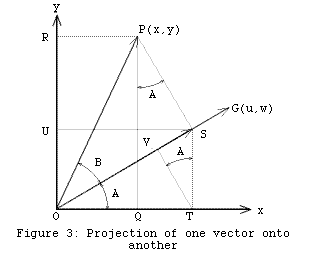If you want to know the x-coordinate of a point like P in Figure 3, you draw a line through P at right angles to the x-axis, and see where it hits the axis. In the process, the vector OP is shown to be the sum of two vectors: OQ, which is parallel to the x-axis, and QP, which is perpendicular to it.

The same thing can be done with any other vector in place of the x-axis. If a line from P to OG meets OG at a right angle, at point S, then OS is called the projection of OP onto OG. And again, OP is shown to be the sum of two vectors: OS, which is parallel to OG, and SP, which is perpendicular.

How long is OS? If the angle between OP and OG is B:

 OS = OP cos B = |(x,y)| cos B (5)

What if we don’t know B? Suppose all we know are the coordinates of P, (x,y), and the angle A that OG makes with the x-axis. Projecting OS onto the coordinate axes to make OT and OU, and projecting OT back onto OS to make OV:

 OS = OV + VS = OT cos A + OU sin A = g[(OT, OU),(cos A, sin A)]

We don’t know (OT, OU), but we do know that it’s the part of the vector (x,y) parallel to OG, when (x,y) is written as a sum of parallel and perpendicular components:

 (x,y) = (OQ, OR) = (OT, OU) + (–QT, UR)

Making use of Eqn (2c):

 g[(x,y),(cos A, sin A)] = g[(OT, OU),(cos A, sin A)] + g[(–QT, UR),(cos A, sin A)] = OS + (–QT cos A + UR sin A) = OS + (–PS sin A cos A + PS cos A sin A) = OS
 OS = g[(x,y),(cos A, sin A)] (6)

What’s this vector (cos A, sin A)? It points in the same direction as the vector OG, but from what we know about sine and cosine:

 |(cos A, sin A)| = √((cos A)2 + (sin A)2) = 1

A vector like this, with a magnitude of one, is known as a unit vector. Eqn (6) tells us that to calculate the length of the projection of (x,y) onto OG, we just apply the metric function to (x,y) and the unit vector in the direction of OG.

What if we don’t know the angle A, but only the coordinates of G, (u,w)? We can still compute the unit vector in this direction, just by dividing (u,w) by its own length, automatically re-scaling it to a length of one.

 (cos A, sin A) = (u,w) / |(u,w)| OS = g[(x,y),(cos A, sin A)] = g[(x,y),(u,w)/|(u,w)|] = g[(x,y),(u,w)] / |(u,w)| (7)

Here we’ve made use of Eqn (2b) to shift the factor 1/|(u,w)| outside the metric.

Equating the two formulas for OS from Eqns (5) & (7) gives:

 |(x,y)| cos B = g[(x,y),(u,w)] / |(u,w)| cos B = g[(x,y),(u,w)] / (|(x,y)||(u,w)|) (8a) g[(x,y),(u,w)] = |(x,y)||(u,w)| cos B (8b)

Eqn (8a) is identical to Eqn (4), which is what we set out to prove.

Setting B equal to 90°, Eqn (8b) shows that the metric function for two perpendicular vectors is zero, since the cosine of 90° is zero. That’s why the metric is able to “pick out” the part of one vector that’s parallel to another; the part that’s perpendicular simply yields zero.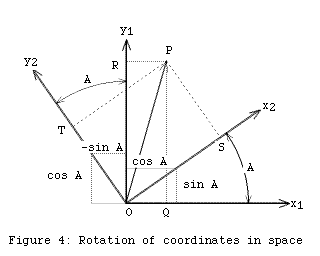Calculating the way a point’s coordinates change when the reference frame is rotated in space is easy, now. In Figure 4, imagine you’re standing at the origin, O, looking straight ahead in the direction of the axis marked y1, with x1 pointing directly to your right. P marks some fixed object in front of you, say a tree. OQ measures how far to the right of you the tree is, and OR measures how far ahead it is. (Negative numbers would be used if it was to the left, or behind you.)

Now suppose you turn your entire body through the angle A, so you’re looking in the direction y2, and x2 points directly to your right. The new coordinates you’d give P are OS and OT. We already have OS for exactly this situation, Eqn (6), and all that’s needed to work out OT are the coordinates of a unit vector that points along the y2 axis. From Figure 4 it’s clear that (–sin A, cos A) does the job, so:

 OT = g[(OQ, OR),(–sin A, cos A)] (9)

Writing (x1,y1) for the coordinates of any point in the original reference frame, and (x2,y2) for the coordinates of the same point in the rotated reference frame, Eqns (6) and (9) become:

 x2 = g[(x1,y1),(cos A, sin A)] = x1cos A + y1sin A (10a) y2 = g[(x1,y1),(–sin A, cos A)] = y1cos A – x1sin A (10b)

This is the standard way of expressing the change of coordinates for a rotation in space. There’s another way, though, which is worth writing down because of its similarity with the most common form of the equivalent spacetime equations. Put

 s = tan A = sin A / cos A

Then s is just the slope of the x2 axis as a line in the (x1,y1) reference frame, and the vector (1,s) points along the x2 axis, while the vector (–s,1) points along the y2 axis. These are not unit vectors, but we can apply Eqn (7) and divide out their lengths:

 x2 = g[(x1,y1),(1,s)] / |(1,s)| = (x1 + sy1) / √(1 + s2) (11a) y2 = g[(x1,y1),(–s,1)] / |(–s,1)| = (y1 – sx1) / √(1 + s2) (11b)

### Spacetime Geometry

The fact that spacetime has four dimensions, as opposed to the three of space alone, is important, but it’s far from being the distinguishing feature of spacetime geometry. Our simple problem in interstellar travel involves only one dimension of space and one of time — a two-dimensional “slice” through four-dimensional spacetime — but the geometry that applies to that slice is not the same as the geometry of the familiar two-dimensional Euclidean plane.In Euclidean geometry, given two fixed points, the (x,y) coordinates you give those points will depend on the reference frame you choose. The coordinates you give P and Q in Figure 5 depend on where you stand, and the direction you’re facing. But the distance between the points, PQ, which can be calculated with Pythagoras’s theorem, must always be the same. A quantity like this, which every observer agrees on, is called an invariant. That the distance between points is an invariant in Euclidean geometry seems almost too obvious to mention, but it’s worth checking that both Eqns (10) and (11) yield the result:

 x22 + y22 = x12 + y12

Points in spacetime are usually called events, to distinguish them from points in space. Events can be specified by giving their space and time coordinates, (x,t), according to a particular observer. For example, in Figure 1, if the event of the spacecraft’s launch is (0, 0), the event of its arrival would be (8.7, 14.5).

The question is, is there a “spacetime distance” between these two events, which everyone can agree on? Is there an invariant in spacetime geometry equivalent to the Euclidean concept of distance?

In the 1880s, Michelson and Morley carried out a series of experiments which established that the speed of light in a vacuum is always the same, regardless of any motion of either the source of the light or the observer making the measurement. Light travels along paths that cut across spacetime in such a way that everyone agrees on its speed. This is the fact that Einstein used to uncover the geometry of spacetime.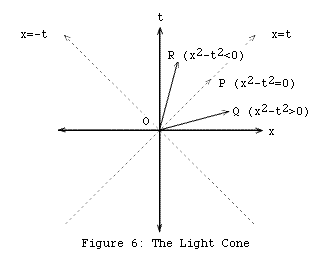Imagine the set of world lines traced out in spacetime by all the pulses of light, travelling in every possible direction, that could pass through a given event. This is known as the light cone for that event. If we’re only dealing with one dimension of space, “every possible direction” means either left-to-right or right-to-left, as illustrated by the two dashed 45° lines in Figure 6, but if you imagine spinning this diagram around the t-axis, you’ll see what the case for two spatial dimensions looks like, and why the term “light cone” is used.

In this diagram, as in Figure 1, we’ve chosen units where the speed of light (normally referred to as c) is one. In everyday units, c = 300,000 km/sec, but using light years and years (or any similar choice, like light minutes and minutes) conveniently makes c = 1. Be warned, though: if you plug distances and times in metres and seconds into any of the formulas we’re about to derive, they won’t work.

Given a velocity of one, the equations for a pulse of light travelling left-to-right or right-to-left through the event (0,0) are:

 x = t x = –t

These two cases can be encompassed by a single equation for the whole light cone:

 x2 – t2 = 0 (12)

The Michelson-Morley experiments showed that, no matter what your own velocity is, you will agree that this is the equation for the light cone. So for any two events whose separation in spacetime is such that a pulse of light can travel between them, such as O and P in Figure 6, the quantity x2t2 must be zero — whoever calculates it, and no matter what individual x and t values they measure.

That makes x2t2 a good candidate to take the place in spacetime of x2+y2 in space. A small change to Eqns (1) and (3) can accommodate this:

 g[(x,t),(u,w)] = xu – tw (13) |(x,t)|2 = g[(x,t),(x,t)] = x2–t2 (14a, if x2–t2 > 0) |(x,t)|2 = –g[(x,t),(x,t)] = t2–x2 (14b, if x2–t2 < 0) |(x,t)|2 = 0 (14c, if x2–t2 = 0)

The new formula for g given in Eqn (13) is known as the Minkowskian, or “flat spacetime”, metric, as opposed to the Euclidean metric of Eqn (1). This metric meets the same conditions of symmetry and linearity as the Euclidean metric, spelt out in Eqns (2).

From Figure 6, it’s clear that some vectors in spacetime, such as OR, can have negative values for x2t2, so the new equivalent of Pythagoras’s theorem, Eqns (14), need to take account of this possibility. Although it might seem a shame to have to divide vectors in spacetime into different classes and treat them each somewhat differently, the three possibilities involve very real physical distinctions, so it’s a good idea not to try to gloss over the differences.

If x2t2 > 0, the vector (x,t) is called spacelike. A spacelike vector slopes away from the time axis at a greater angle (and hence a greater velocity) than a light ray. No object’s world line can point in a spacelike direction. In Figure 6, not even a pulse of light at event O can travel fast enough to reach event Q. For events with spacelike separation, |(x,t)| is called the proper distance between them; an observer who judges them to have happened simultaneously measures t = 0, so |(x,t)| = x.

If x2t2 < 0, the vector (x,t) is called timelike. A timelike vector slopes away from the time axis at a smaller angle than a light ray. Ordinary objects’ world lines point in timelike directions. In Figure 6, there’s nothing to stop a spacecraft cruising from event O to event R — and an observer on the spacecraft would consider that the two events were separated only in time, not space. For events with timelike separation, |(x,t)| is called the proper time between them; an observer who judges them to have happened at the same place measures x = 0, so |(x,t)| = t.

If x2t2 = 0, the vector (x,t) is called lightlike, or null — because the vector’s length is zero, however large its individual x and t components are. Only photons, the particles of light (and other massless particles) have world lines pointing in null directions.

The light cone is a real, physical structure, not an artifact of the coordinates you happen to choose. And since it marks the division between timelike vectors and spacelike ones, every observer will assign a given vector to the same class. Motionless versus stationary is a matter of opinion. Timelike versus spacelike is not.

To convert between spacetime coordinates for different observers, we’ll need to be able to project one spacetime vector onto another. It would be nice to be able to use the equations we’ve established for vectors in space, like Eqn (7), and simply substitute the new metric. But rather than doing that blindly, we need to take a closer look at what the idea of projection really means, in spacetime.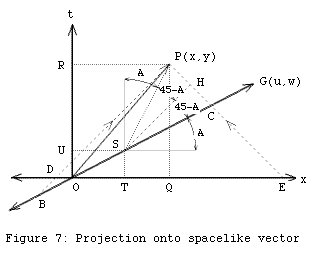Suppose we want to determine the x-coordinate of the event P in Figure 7. We want to pin down its location in space, by marking it on the x-axis. In space alone, we’d do this simply by drawing a line through P at right angles to the x-axis — but is there any justification for doing this on a spacetime diagram?

DP and EP are the world lines of two pulses of light, aimed at each other, which collide precisely at event P. Assuming that the two pulses leave “at the same time”, they must travel equal distances in order to arrive together. So the event Q, mid-way between D and E, marks P’s location on the x-axis. Because the light rays DP and EP both make 45° angles with the x-axis, QP must be perpendicular to the axis.

But now suppose we use the same method to project OP onto some arbitrary spacelike vector OG, instead of the x-axis. The same two light rays intersect OG at B and C, and the event S is mid-way between them. So according to an observer who considers that B and C (rather than D and E) happen “at the same time”, S and P (rather than Q and P) happen “at the same place”. The two concepts are bound together, just like “left-right” and “forwards-backwards”; exactly what you mean by each one depends on what you mean by the other.

OS is the projection of OP onto OG, in exactly the same sense as OQ is the projection of OP onto the x-axis. Does the spacetime metric, Eqn (13), give us the length of OS? First, since SP isn’t perpendicular to OG, it will help to know what its direction is. By drawing in another 45° line, SH, parallel to BP, we can make a triangle SHC, the same shape as BPC but half the size (since BS and SC are equal). That means H must bisect PC, and the angles either side of it will be equal.

SP, then, must make exactly the same angle with the t-axis as OG does with the x-axis. Since OG is the vector (u,w), and SP has coordinates (TQ, UR):

 TQ/UR = tan A = w/u TQ u = UR w TQ u – UR w = 0 g[(TQ, UR),(u,w)] = 0

Applying the Euclidean metric to perpendicular vectors in Euclidean space gives zero. By the same criterion, this shows that SP and OG really are perpendicular, in spacetime, even though the lines we draw for them on paper are not. In a perspective drawing of a room where a certain wall is viewed face-on, right angles on that wall will be right angles in the drawing — but right angles on the floor, the ceiling, and other walls will not. Don’t take this analogy too seriously — the details in each case are quite different — but when you’re drawing a spacetime diagram it would be a great surprise if you could show everything without distortion.

Now, to find OS, we make use of Eqn (14a), the new version of Pythagoras’s theorem:

 OS2 = OT2 – OU2 OS = OT (OT/OS) – OU (OU/OS) = g[(OT, OU),(OT/OS, OU/OS)]

and note that (OT/OS, OU/OS) is a unit vector:

 |(OT/OS, OU/OS)| = (OT/OS)2 – (OU/OS)2 = (OT2 – OU2) / OS2 = 1

As in Euclidean space, we can find the unit vector in the direction of OG by dividing (u,w) by its length:

 (OT/OS, OU/OS) = (u,w) / |(u,w)|

and so:

 OS = g[(OT, OU),(u,w)] / |(u,w)|

Now, we know that:

 (x,y) = (OQ, OR) = (OT, OU) + (TQ, UR)

Making use of Eqn (2c):

 g[(x,y),(u,w)] / |(u,w)| = g[(OT, OU),(u,w)] / |(u,w)| + g[(TQ, UR),(u,w)] / |(u,w)| = OS + 0 = OS

We’ve shown that the spacetime metric gives the correct length of the projection OS, where S is the point on OG mid-way between two light rays that converge on P. But we’ve assumed that OG is spacelike. What about projections onto the t-axis, and other timelike vectors? Two pulses of light that leave the t-axis at different moments will never even meet — the second one will never catch up with the first. For timelike vectors, we need to take a slightly different approach.Suppose that instead of arranging for two pulses of light to collide at P, we imagine bouncing a radar pulse off some object at P, and waiting for the reflection to come back. The time the reflection occurs will then be exactly halfway between the time the pulse was sent out and the time it returns. In Figure 8, a pulse leaves the t-axis at D, and returns at E, so R, mid-way between D and E, must mark P’s time coordinate.

As with the projection onto the x-axis, RP is at right angles to the t-axis. But if we apply the same method to another timelike vector, OG, the same radar pulses would be observed leaving and returning at B and C. B and C happen “at the same place”, according to an observer whose world line points in the direction OG, so the event S, mid-way between B and C, must happen “at the same time” as P according to that observer — just as R happens “at the same time” as P for any observer whose world line is parallel to the t-axis.

Again, SP isn’t perpendicular to OG in the normal Euclidean sense, when we draw it on paper. But the same construction shows that it’s perpendicular in the spacetime sense. The only difference comes when we calculate the length of OS; because OG and OS are timelike vectors, we have to use Eqn (14b) instead of (14a):

 OS2 = OU2 – OT2 OS = OU (OU / OS) – OT (OT / OS) = –g[(OT, OU),(OT/OS, OU/OS)] = –g[(OT, OU),(u,w)] / |(u,w)|

This means there are two slightly different equations for the length of the projection of (x,t) onto (u,w), depending on whether (u,w) is spacelike or timelike. (If (u,w) is lightlike, then the length of the projection is always just zero.)

 length of projection = g[(x,t),(u,w)] / |(u,w)| (15a, if u2–w2 > 0) length of projection = –g[(x,t),(u,w)] / |(u,w)| (15b, if u2–w2 < 0)

### Rotations in Spacetime

Now, imagine a ship that happens to be cruising past the Earth with velocity v. The event of the ship’s closest approach to the Earth is taken to be the origin of spacetime coordinates, for both an observer on Earth and an observer on the ship. But they differ on the direction of the time and space axes, just as the directions “left-right” and “forwards-backwards” are different for the two coordinate systems in Figure 4.Since the ship is cruising past the Earth with velocity v, an observer on Earth would consider the ship’s world line to be:

 x1 = vt1 (16a)

while an observer on the ship itself considers it to be stationary:

 x2 = 0 (16b)

This is just the t2 axis. So on a spacetime diagram drawn by the Earth observer, such as Figure 9, the t2 axis is given by Eqn (16a). What’s more, since (x1,t1) = (v,1) solves Eqn (16a), (v,1) is a vector pointing along the t2 axis.

The x2 axis must be perpendicular to the t2 axis, in the spacetime sense. It’s easy to see that (x1,t1) = (1,v) points in the right direction:

 g[(1,v),(v,1)] = v – v = 0

which means the x2 axis, in Earth and ship coordinates, is:

 t1 = vx1 (17a) t2 = 0 (17b)

The shipboard observer’s idea of the things that are happening “right now” at time zero isn’t the same as the Earth observer’s: the two x-axes in Figure 9 don’t coincide, and the further you move away from the origin, the further apart they become. But this is no different from the situation where two people, standing in the same location but facing different directions, fail to agree as to which objects are “precisely to the right”, and their disagreement is greater for objects a kilometre away than a metre away. Our bodies carry the definitions of left-right, forwards-backwards, and up-down with them. What Einstein showed is that we also carry our own definition of the direction “future-past”, perpendicular to the other three. Relative motion means that two peoples’ future-past axes point in different directions in spacetime, and it’s as unreasonable to expect their idea of “to my left, but no earlier or later” to match up under those circumstances as it is to expect two people facing north and north-east to mean the same thing by the phrase “to my left, but not forwards or backwards”.

We now have everything we need to write down the equations for the change of coordinates between the Earth’s reference frame and the ship’s. Using Eqns (15a) and (15b) in turn to project the vector with Earth coordinates (x1,t1) onto, first, the spacelike vector (1,v) which points along the x2 axis, and then the timelike vector (v,1) which points along the t2 axis:

 x2 = g[(x1,t1),(1,v)] / |(1,v)| = (x1 – vt1) / √(1 – v2) (18a) t2 = –g[(x1,t1),(v,1)] / |(v,1)| = (t1 – vx1) / √(1 – v2) (18b)

These equations are called a Lorentz transformation, or to be more specific, a boost. (Lorentz transformations also include completely general rotations in four-dimensional spacetime, which might include some rotation in space.)

The reverse transformation, from ship coordinates (x2,t2) to Earth coordinates (x1,t1), is identical to Eqns (18), except that v is replaced by –v, since from the ship’s point of view the Earth appears to be travelling in the opposite direction.

 x1 = g[(x2,t2),(1,–v)] / |(1,–v)| = (x2 + vt2) / √(1 – v2) (19a) t1 = –g[(x2,t2),(–v,1)] / |(–v,1)| = (t2 + vx2) / √(1 – v2) (19b)

As you’d expect, it follows from either Eqns (18) or (19) that:

 x22 – t22 = x12 – t12

Whatever the Earth-based and shipboard observers disagree on, for a given event they always calculate the same value for x2t2.

### Time Dilation

Suppose a man and a woman are walking on the surface of the Earth (over short enough distances for curvature to be negligible). They start out together, but the man walks due north, and the woman walks north-east, as in Figure 10.

By the time the man reaches point B, the woman would have to have travelled much farther, all the way to point E, to have reached the same latitude and be precisely to the man’s right. But … so what? By the time the woman reached point D, the man would have to have travelled all the way to point C to have gone as far in the north-east direction as the woman, and be precisely to her left. Each might think that the other has to “run fast” in order to “keep up”, but their situation is completely symmetrical.Time dilation is the spacetime equivalent of this scenario. The length of a path in spacetime is the time that has elapsed along that path, and because the spacetime version of Pythagoras’s theorem has a minus sign, the time that elapses along what looks like the longer path is actually less, not more.

Figure 11 shows the world lines of two astronauts, a man spacewalking in Earth orbit, and a woman tethered to a ship cruising past with velocity v. At event A, they pass within waving distance and synchronise their watches.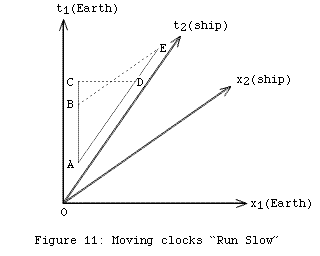Despite appearances to the contrary — due to the distortions of drawing spacetime on a piece of paper — the time that elapses for the man from A to C is exactly the same as the time that elapses for the woman from A to E. The proper time, √(t2x2), is the same in both cases. And while CD is obviously perpendicular to the man’s world line, BE is equally perpendicular to the woman’s world line, since it’s parallel to the x2 axis.

Suppose the man’s watch records 10 seconds elapsing from A to C, and the woman’s watch records an equal time elapsing from A to E. At C, the man considers the woman to have reached only event D, so less time must have passed for her: her watch must be “running slow”. But conversely, at E the woman considers the man to have reached only event B, so less time must have passed for him, and his watch must be running slow. Again, the situation is perfectly symmetrical.

Of course, neither can see the other’s watch immediately — assuming they can see it at all, with binoculars or whatever — so in practice they could only figure out these relationships after the fact: by keeping a record of their observations and then subtracting out the time it took the light to reach them. But time dilation has nothing to do with these time lags; the effect involves the situation deduced by each astronaut after taking account of time lags.

Eqn (18b) is:

 t2 = (t1 – vx1) / √(1 – v2)

Given that the man measures no separation in space, x1, between A and B, this becomes:

 AE = AB / √(1 – v2) AB = AE √(1 – v2)

For example, if AE is 10 seconds, and v = 0.6 (60% of lightspeed), then:

 AB = 10 √(1 – (0.6)2) = 10 √(1 – 0.36) = 10 √(0.64) = 8 seconds

AB is the time the woman concludes has elapsed for the man, when she’s reached event E and 10 seconds has passed for her. By symmetry, AD (the time the man concludes has elapsed for the woman, when 10 seconds has passed for him) is also 8 seconds. Both astronauts are correct, they’re just talking about different things.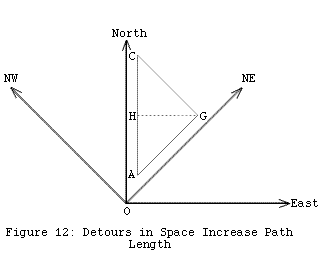With all this symmetry, you might be starting to wonder how space travellers can ever return home younger than their twins. What makes this possible is the fact that a two-way voyage is not symmetrical; however you look at it, the space travelling twin takes a detour from the straight-line path of the twin who stays at home.

The analogy in space is completely familiar. In Figure 12, the shortest path from A to C is the straight line that happens to run due north. If a man and a woman set off from A, and the man travels due north, but the woman travels north-east for the first half of the trip and north-west for the second half, it’s obvious that when the two meet up at C, the woman will have travelled farther. AG and GC are both longer than AH and HC:

 AG = √(AH2 + HG2) > AH GC = √(HC2 + HG2) > HC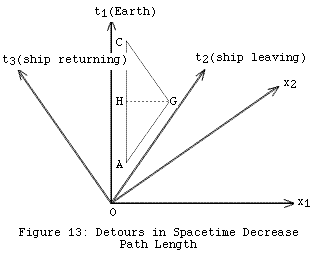In Figure 13, when the astronaut tethered to the ship reaches G, the ship reverses and heads back towards the Earth. (In reality, a perfectly sharp turn like this would require infinite acceleration, but to simplify the analysis we’ll ignore any actual rounding of the corner at G.) The situation looks exactly the same as Figure 12, but now AG and GC are shorter than AH and HC:

 AG = √(AH2 – HG2) < AH GC = √(HC2 – HG2) < HC

We can quantify this, by noting that if the ship’s velocity is v for both stages:

 HG = v AH AG = √(AH2 – v2 AH2) = AH √(1–v2) HG = v HC GC = √(HC2 – v2 HC2) = HC √(1–v2) (AG + GC) = AC √(1–v2)

After synchronising watches at A, when the woman passes the man for the second time, at event C, her watch shows less elapsed time than the man’s. In spacetime, a straight world line is the longest path between two events, not the shortest.

### The Flight to Sirius

To calculate how much time passes on board the spacecraft travelling to Sirius in Figure 1, consider each stage of the journey separately. The middle stage is easy:

 ship time (cruising) = √((3.5)2 – (2.9)2) = 1.96 years

To analyse the acceleration stage, we need to know the exact shape of the curved world line. To determine this with complete mathematical rigour takes a bit of work, but there’s a nice intuitive way to reach the same answer. In classical mechanics, the distance travelled in time t by a uniformly accelerating object starting from rest is:

 x = at2 / 2

(Why? Because its final velocity is v = at, but it started out with v = 0, so its average speed is halfway in between, v = at/2.) Given x and t, the rate of acceleration is:

 a = 2x / t2

The same relationship holds in relativistic mechanics, but t is replaced by the proper time, √(t2x2), measured along a straight line in spacetime between the endpoints of the curved world line:

 a = 2x1 / (t12 – x12) (20a)

Acceleration here must be measured in units consistent with the speed of light being one, such as light-years/year2. In those units, one Earth gravity is:

 9.8 metres/sec2 = 9.8 × 3600 × 24 × 365 / 300,000,000 light-years/year2 = 1.03 light-years/year2

Unfortunately, there’s no easy shortcut for computing the proper time along the curved world line, which is the elapsed ship time. This is given by:

 ship time (accelerating) = ln (1 + a (x1 + t1)) / a (20b)

In Figure 1, the craft travels 2.9 light years in 5.5 years, so Eqn (20a) gives:

 a = 2 × 2.9 / ((5.5)2 – (2.9)2) = 0.266 light-years/year2

and Eqn (20b) gives:

 ship time (accelerating) = ln (1 + 0.266 × (2.9 + 5.5)) / 0.266 = 4.41 years

By symmetry, the deceleration stage takes exactly the same time, so the total shipboard time for the journey is 4.41 + 1.96 + 4.41 = 10.78 years, compared to 14.5 years Earth or Sirius time.

### Doppler Shift and Aberration

A beam of light can be thought of as consisting of a series of evenly spaced wavefronts in spacetime. (This doesn’t begin to do justice to the physics of electromagnetic waves, but it captures the essential geometry of the situation.) In four-dimensional spacetime, these wavefronts are three-dimensional hyperplanes, but since we’re only going to tackle problems involving one or two dimensions of space, wavefronts will appear either as lines in two-dimensional spacetime (Figure 14) or planes in three-dimensional spacetime (Figure 15).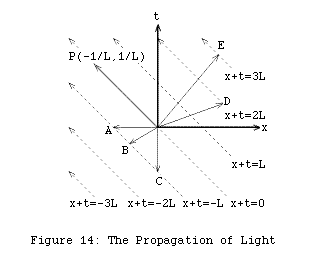Figure 14 shows light travelling right-to-left through one-dimensional space; the wavefronts are all inclined at 45° in spacetime. If the t-axis was the world line for your eye, it would be struck by a wavefront at regular intervals; the number of times this happens per second is called the frequency of the light, and the time between wavefronts is called the period. The distance between wavefronts in space is called the wavelength; in units such that the speed of light is one, the wavelength and the period will be equal.

The direction and spacing of a series of wavefronts like this can be described by a single spacetime vector, called the propagation vector, parallel to the wavefronts’ world lines. Both the space and time components of the propagation vector are given a length of 1/L, where L is the wavelength of the light, and the space component points in the direction the light is travelling. In the one-dimensional example of Figure 14, that just means the x coordinate has a minus sign to indicate right-to-left, so the propagation vector is (–1/L,1/L).

The propagation vector as a whole has length zero, of course — whatever its individual space and time components — since it points in a lightlike direction in spacetime.

 |(–1/L,1/L)|2 = 1/L2 – 1/L2 = 0

If you draw a vector from the origin to any one of the wavefronts, the propagation vector provides a simple way to determine, mathematically, which wavefront each vector is touching. The lines for the wavefronts in Figure 14 all take the form:

 x + t = nL

where n is an integer. So events on these wavefronts have (x,t) coordinates (x, nLx). The spacetime metric operating on the propagation vector and one of these vectors gives:

 g[(–1/L, 1/L),(x, nL–x)] = –x/L – n + x/L = –n

which identifies the wavefront with a number that’s completely independent of the particular vector you chose. So in Figure 14, without going to the trouble of working out the coordinates of points A to E, we know that:

 g[P, A] = 1 g[P, B] = 1 g[P, C] = 1 g[P, D] = –2 g[P, E] = –3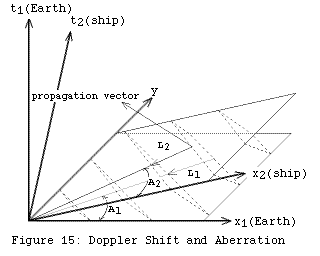Figure 15 shows a series of planar wavefronts, moving through the coordinate systems of two observers in relative motion. The two planes defined by either the x1 axis or the x2 axis and the shared y axis are the slices through three-dimensional spacetime that Earth-based and shipboard observers consider to be “space, at time zero.” Because these spacelike slices cut through the wavefronts at different angles, both the angle of approach and the spacing between the wavefronts — the wavelength of the light — will be different for the two observers.

To quantify this, suppose the shipboard observer sees starlight with wavelength L2, at an angle A2 from the direction of travel — anything from 0° for a star that’s straight ahead to 180° for a star directly behind the ship. (The second angle needed to pin down most stars, the angle measured around the ship’s axis, makes no difference to the analysis.) The propagation vector according to the shipboard observer will be:

 (x2,y,t2) = (–cos A2, –sin A2, 1) / L2 (21)

since the space component of this, (x2,y), points in the right direction — towards the ship, at an angle A2 — and both the space and time components taken individually have length 1/L2. (The length of the space component is just |(x2,y)|, in the usual Euclidean sense.)

Using Eqns (19) — leaving the y coordinate unchanged — we can transform this vector into Earth-based coordinates:

 x1 = (v – cos A2) / (L2 √(1–v2)) (22a) y = –sin A2 / L2 (22b) t1 = (1 – v cos A2) / (L2 √(1–v2)) (22c)

But the time and space components of the propagation vector in Earth coordinates must both have length 1/L1, where L1 is the wavelength measured by an Earth observer. The single time component is easiest to deal with:

 1/L1 = (1 – v cos A2) / (L2 √(1–v2)) L2/L1 = (1 – v cos A2) / √(1–v2) (23)

Eqn (23) describes the Doppler shift, the difference in the wavelength of light as measured by two observers in relative motion. For A2 = 0° and 180°, respectively:

 L2/L1 = (1–v) / √(1–v2) = √((1–v)/(1+v)) L2/L1 = (1+v) / √(1–v2) = √((1+v)/(1–v))

Assuming v is positive, starlight from straight ahead always has a shorter wavelength — a blue shift — and starlight from behind always has a longer wavelength — a red shift. The angle at which the crossover occurs — the direction in which the wavelength is unaltered — depends on the velocity:

 1 = (1 – v cos A2) / √(1–v2) 1 – v cos A2 = √(1–v2) cos A2 = (1 – √(1–v2)) / v

 velocity L2/L1 for A2=0 A2 for L2 = L1 0.5 0.577 74.5° 0.7 0.420 65.9° 0.9 0.229 51.2°

For a ship travelling at 90% of lightspeed, the wavelength of starlight from straight ahead is less than a quarter the usual value. This is enough to shift all visible light into the ultraviolet (though it also shifts a band of infrared wavelengths into the visible spectrum, so stars won’t necessarily vanish from sight). Looking back, the effect is reversed: wavelengths are multiplied by a factor of more than four, turning all visible light into infrared (and rendering some UV visible).

For angles in between, the Doppler shift varies smoothly, ringing the spacecraft with bands of stars shifted by different amounts — the famous “starbow” of interstellar travel. Individual stars are different colours anyway, so all the stars at a given angle won’t look identical, but the average colour of the sky will be graded like a circular rainbow, positioned roughly at the crossover angle, 50° in this case.

Eqns (22) for the propagation vector also show that the direction the wavefronts move within the (x1,y) plane is:

 (x1,y) = ((v – cos A2) / (L2 √(1–v2)), –sin A2 / L2)

If the wavefront is seen by an Earth-based observer to approach at an angle A1 from the x1 axis, the ratio of y- to x1-coordinates for this vector must equal tan A1:

 tan A1 = sin A2 √(1–v2) / (cos A2 – v) (24)

Eqn (24) describes an effect known as aberration. For travellers moving at a substantial fraction of lightspeed, the familiar constellations will appear to have been pushed forward in the sky, crowded around the direction in which the ship is travelling.

To take two examples, for stars that appear to a stationary observer to be 90° away from the ship’s destination:

 cos A2 = v

and for stars that appear to a moving observer to be 90° away from the same point:

 tan A1 = –√(1–v2) / v

 velocity A2 for A1 = 90° A1 for A2 = 90° 0.5 60.0° 120.0° 0.7 45.6° 134.4° 0.9 25.8° 154.2°

For a ship travelling at 90% of lightspeed, the constellations that would normally have occupied the entire forward hemisphere are squashed together into a circle 25° in radius. Looking backwards, the stars that would have been confined to a 25° circle in the stationary view are spread out to fill half the sky.Foundations / 1: Special Relativity / created Thursday, 11 June 1998 / revised Saturday, 20 June 1998